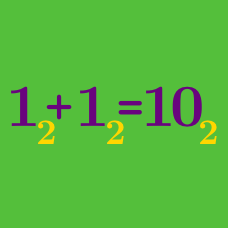Number Theory

# Number Bases: Level 3 Challenges

There is a number $$a$$, which can be written as $$\overline{xyz}$$ in base 9 and $$\overline{zyx}$$ in base 6, for some positive integer $$x,y,z$$. Find $$x+y+z$$.

Details and Assumption

$$\overline{xyz}$$ represents reading the digits together, instead of multiplying them out. For example, $$\overline{xyz}_9 = 81 x + 9y + z$$ and $$\overline{zyx}_6 = 36 z + 6y + x$$.

Find the smallest natural number greater than 3 which has the unit digit of 3 when expressed in base-4, base-5, base-6, base-7, and base-8.

$$N$$ is an integer whose representation in base $$b$$ is 777. Find the smallest positive integer $$b$$ for which $$N$$ is the fourth power of an integer.

$(2^3)! = 40320_{10} = 1001\:1101\:1000\:0000_2.$

The factorial shown above has seven trailing zeros.

How many trailing zeroes does the number $$(2^{16})!$$ have in binary notation?

$1,3,4,9,10,12,13,\ldots$

The above sequence consists of the powers of $$3$$ or the sum of distinct powers of $$3$$, arranged in increasing order.

Find the $$100^\text{th}$$ term of the sequence.

×